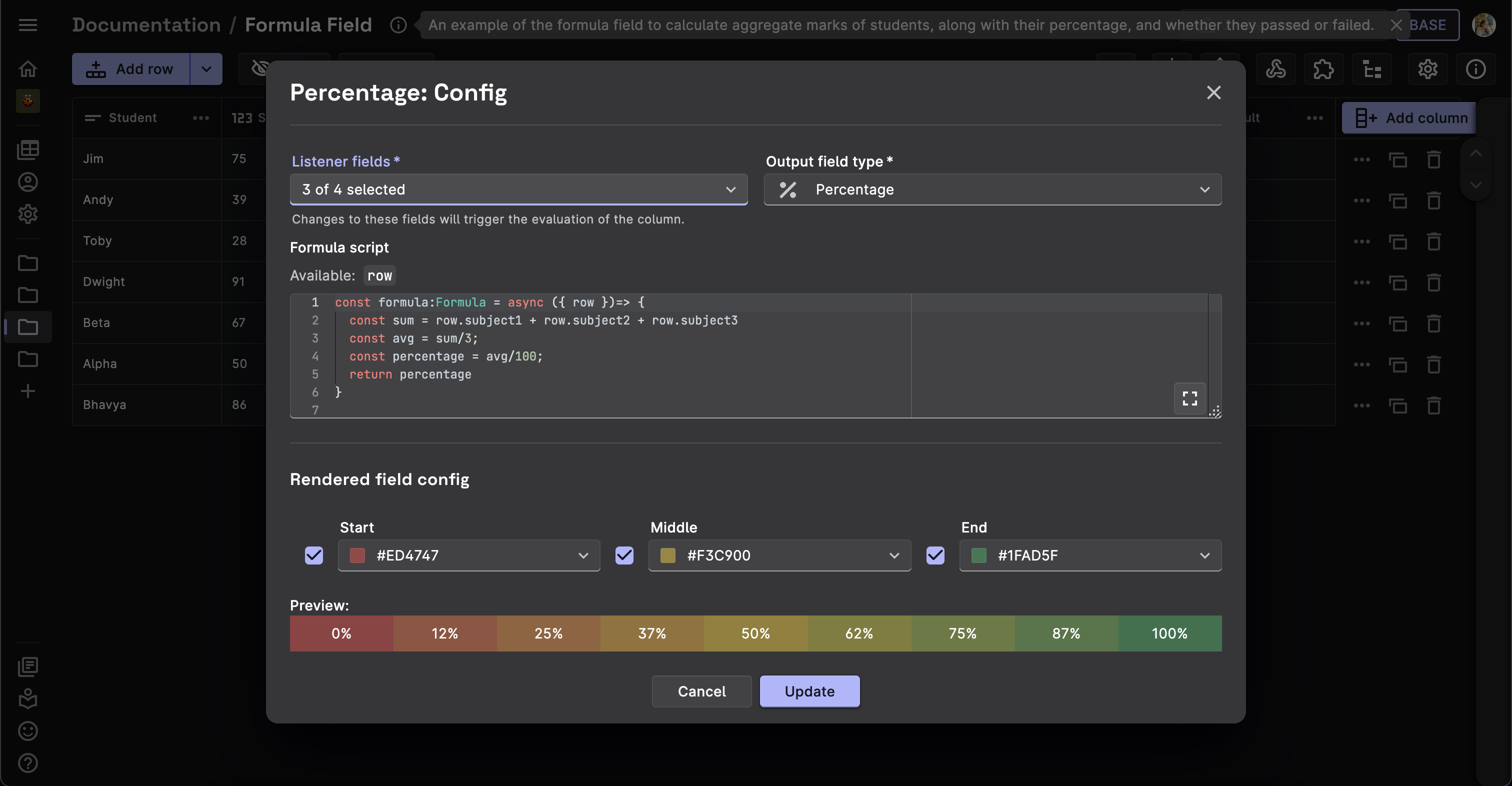# Formula

The Formula field type allows you to operate on the values of the other fields in your table. The formula field enables you to perform calculations such as addition, subtraction, multiplication, division, and more.

In the column config, users can write a function script which includes any mathematical functions, and returns a value.

The Formula field has access to the the `row` parameter, which contains the values of the other fields in the row.##### Formula Field is for UI purposes only

Formula fields are exclusively for UI purposes and are not stored in the database. If you require data to be stored in Firestore, you can utilize the Derivative field. The Derivative Field functions similarly to a formula field but offers greater capabilities. It can persist data to Firestore in real-time, triggered by data events within the table.

## Example code snippets​

Dataset #1: Table contains list of students and their scores in different subjects. Let's see how we can create simple formulae for calculating their average, grade etc.

### Add numbers and find average​

This field will calculate and store the aggregate score of the student.

• Field Type: Formula
• Listener Fields: The three score fields.
• Output Field Type: Number
• Formula:
``const formula:Formula = async ({ row })=> {    const sum = row.subject1 + row.subject2 + row.subject3    const avg = sum/3    return avg;}``

### Percentage​

This field will calculate and store the percentage of the student.

• Field Type: Formula
• Listener Fields: The three score fields.
• Output Field Type: Percentage
• Formula:
``const formula:Formula = async ({ row })=> {    const sum = row.subject1 + row.subject2 + row.subject3    const avg = sum/3;    const percentage = avg/100;    return percentage}``

### If-Then-Else​

This field will calculate and store the grade of the student.

• Field Type: Formula
• Listener Fields: The three score fields.
• Output Field Type: Short Text
• Formula:
``const formula:Formula = async ({ row })=> {    const sum = row.subject1 + row.subject2 + row.subject3    const avg = sum/3;    if(avg>=90){        return "O"    }    else if(avg>=80 && avg<90){        return "A"    }    else if(avg>=70 && avg<80){        return "B"    }    else if(avg>=60 && avg<70){        return "C"    }    else if(avg>=50 && avg<60){        return "D"    }    else if(avg>=40 && avg<50){        return "E"    }    else{        return "F"    }}``

### Check for greater than, less than or equal to​

This is a formula field which will calculate and store the result of the student, whether he passed or failed.

• Field Type: Formula
• Listener Fields: The three score fields.
• Output Field Type: Short Text
• Formula:
``    const formula:Formula = async ({ row })=> {        const avg = (row.subject1 + row.subject2 + row.subject3)/3        return avg >= 40 ? "Pass" : "Fail"    }``

Dataset #2: String Concatenation

Let's see how we can utilize the formula field to concatenate strings.

### Concatenate strings​

This field will concatenate the two strings and store them as a single string.

• Field Type: Formula
• Listener Fields: The two string (Short/Long Text) fields.
• Output Field Type: Long Text
• Formula:
``const formula:Formula = async ({ row })=> {  if(row.string1==undefined && row.string2==undefined){    return "Fields empty!"  }  else if(row.string1==undefined){    return row.string2  }  else if(row.string2==undefined){    return row.string1  }  return row.string1 + " " + row.string2}}``